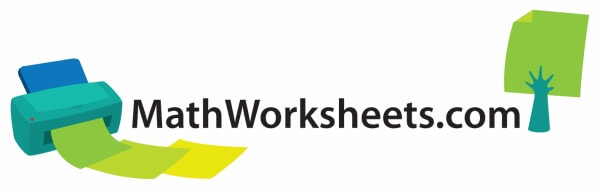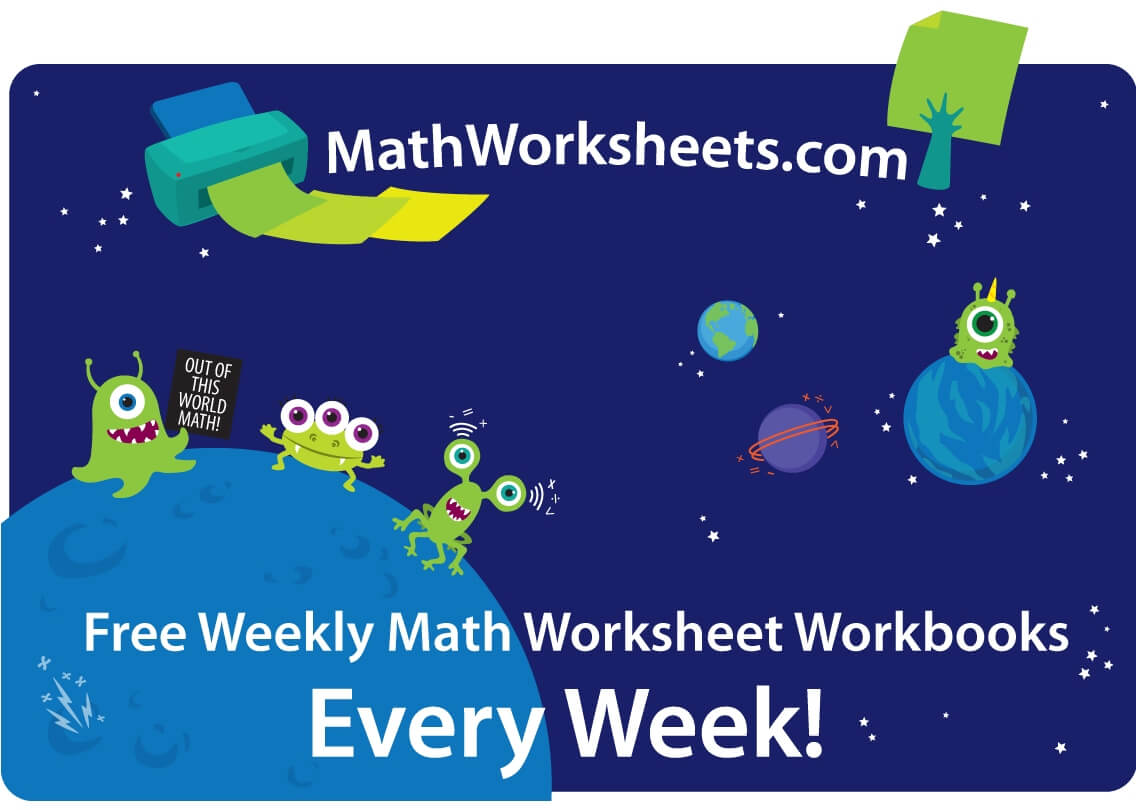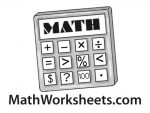## Math Worksheets - Free Weekly PDF PrintablesYour kids from Kindergarten up through sixth grade will love using these math worksheets. New math workbooks are generated each week to make learning in the classroom fun. Word problems are emphasized for a deeper understanding of how math works, along with reinforcing basic math facts. The enrichment math pages will easily complement your existing math program and can be used every week to build the children's math skills and problem-solving strategies. Print as many of these math worksheets as you need to use in the classroom, at home, or at a tutoring center.

Weekly Math Worksheets
Math Worksheets for Week of September 4
Math Worksheets for Week of September 11
Math Worksheets for Week of September 18
Math Worksheets for Week of September 25
Math Worksheets for Week of October 2
Math Worksheets for Week of October 9
Math Worksheets for Week of October 16
Math Worksheets for Week of October 23
Math Worksheets for Week of October 30
Math Worksheets for Week of November 6
Math Worksheets for Week of November 13
Math Worksheets for Week of November 20
Math Worksheets for Week of November 27
Math Worksheets for Week of December 4Addition Division Hundreds Charts